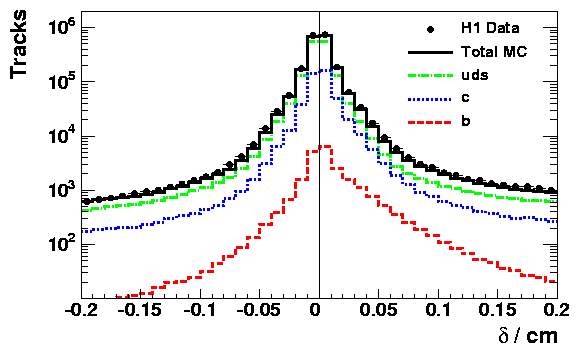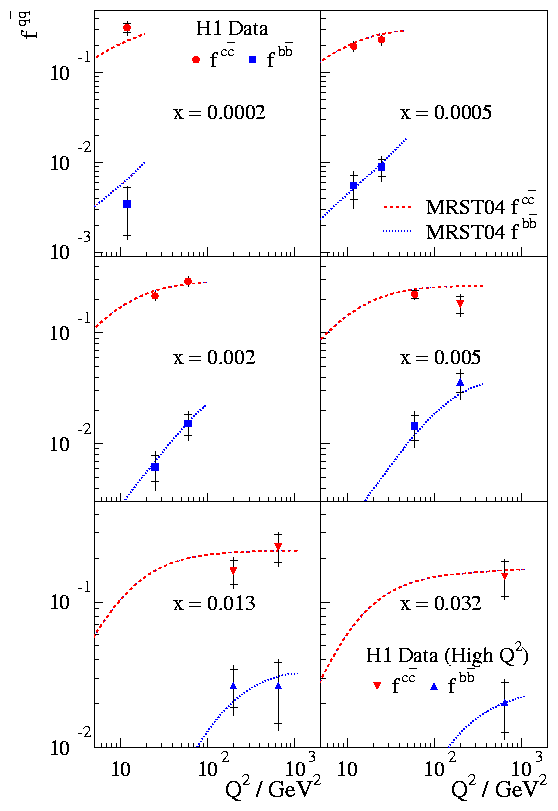# Measurement of F2cc and F2bb at Low Q2 and x using the H1 Vertex Detector at HERA

The above paper is an extension of a previous H1 publication entitled Measurement of F2cc and F2bb at High Q2 using the H1 Vertex Detector at HERA . In summary, the previous paper studied the rate of collisions in which a charm (c) (beauty (b)) quark is produced to provide information on the contribution of c (b) quarks to the proton structure function, called F2cc (F2bb). These heavy quarks do not exist ordinarily in the proton but are produced from the gluon density of the proton. The gluon is the particle which carries the strong force which is responsible for keeping the constituents of matter together. The theory which describes the strong force is called quantum chromodynamics (QCD). The present analysis looked at events in which the resolving power Q2 of photons produced from the electron beam at HERA was much smaller than in the previous analysis. This region is of particular interest because the large mass of the b quark suppresses the phase space available for production. Therefore, the measurement of beauty quarks at low Q2 provides an important test of our understanding of QCD and the strong interaction in the threshold region.

The idea of this analysis was to employ the same experimental techniques to identify c and b quarks as used previously, which are explained in more detail here . The general idea uses the fact that the heavy hadrons (observable particles produced directly from the heavy quarks) have a long lifetime which means they travel some way before decaying to further hadrons.

In order to measure the small distances accurate information from the H1 vertex detector, which is located very close to the interaction point, is used. The device can measure track displacements with accuracies down to 3 hundredths of a mm. In the following figure the distance of charged tracks to the primary vertex is shown. For particles coming from light quarks the average displacement of charged tracks from the primary vertex is zero, within the experimental resolution, leading to a symmetric distribution (green curve). In contrast, particles coming from the decay of heavy hadrons show a positive displacement on average (blue and red curves). The kinematic suppression of the b quark in the region of this measurement means that it is experimentally harder to distinguish c and b quarks from light quarks. However, the asymmetry can still be exploited to unravel the fraction of events coming from c and b quarks in the data.In the kinematic range of the measurement 12 < Q2< 60 GeV2, the decays of heavy flavour hadrons are still very likely to have a track which passes through the H1 vertex detector. Therefore, the charm and beauty structure functions F2cc and F2bb can be reliably measured. This is the first measurement of F2bb at low Q2.

The results for F2cc and F2bb are calculated for three values of Q2 and seven values of x. Here, x is the fraction of the proton's momentum carried by the heavy quark. The results may also be presented in the form of the relative contributions to the total production rate (or cross section) of all quarks. These are shown in the figure below. The values of x are small because the heavy quarks are produced from the "sea" of gluons which holds the proton together. It can be seen that the charm quarks contribute around 20% of the total cross section for all values of Q2. In contrast, the fraction of beauty quarks increase from around 0.4% at Q2 = 12 GeV2 to around 1.5% at Q2 = 60 GeV2. The results are well described by theoretical predictions based on QCD.So what did we learn?

The long lifetime of heavy hadrons compared with light hadrons allows the contribution of heavy quarks to the total production rate of all quarks to be reliably measured. The measurements are made possible by the very precise information obtained by the H1 vertex detector. This is the first measurement of the beauty structure function F2bb at low Q2 and extends into a region where the large mass of the b quark suppresses production. The results are seen to be well described by the predictions from quantum chromodynamics, the theory of the strong interaction.